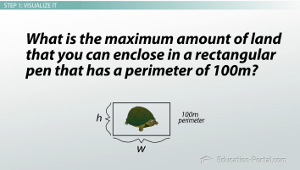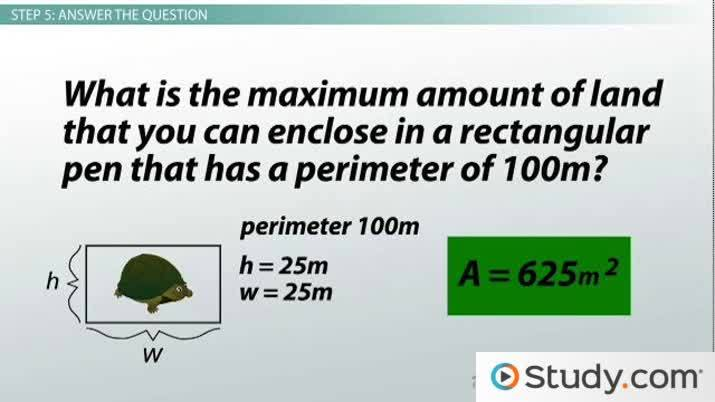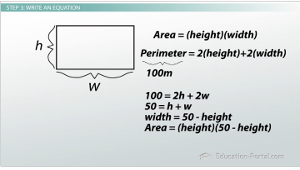Math Courses / Course / Chapter

# Optimizing Simple Systems

Lesson Transcript
Instructor: Sarah Wright
Most optimization problems involving simple systems can be solved using the five-step method. Explore how to solve optimization problems using these five steps: visualizing, defining the problem, writing the equation, finding the minimum or maximum, and answering the question. Updated: 10/24/2021

## Five Steps to Solve Optimization Problems

Remember that optimization problems are everywhere, but we have a five-step way to solve optimization problems. We must visualize the problem, define the problem, write an equation for the problem, find the minimum or maximum for the problem and then answer the question. With these steps, we can solve most optimization problems.Let's try one. What is the maximum amount of land that you can enclose in a rectangular pen that has a perimeter of 100m?An error occurred trying to load this video.

Try refreshing the page, or contact customer support.

Coming up next: Optimizing Complex Systems

### You're on a roll. Keep up the good work!

Replay
Your next lesson will play in 10 seconds
• 0:40 Visualize It
• 1:04 Define the Problem
• 1:21 Write an Equation
• 2:44 Find the Min/Max
• 4:54 Lesson Summary
Save Save

Want to watch this again later?

Timeline
Autoplay
Autoplay
Speed Speed

## Step 1: Visualize It

The first thing we need to do is visualize it. Let's draw this out; I have a rectangular pen. Let's say it's going to hold my turtle (he needs a lot of room). Here's my rectangular pen: it's got a height of h, a width of w and a perimeter of 100m. I don't know what h and w are since they weren't given to me. Instead, I get to pick those to solve this problem.

## Step 2: Define the Problem

Next is the second step, define the problem. I need to maximize the pen area. I'm constrained by my perimeter which has to equal 100m. I don't have any other constraints, so I need to maximize this area which is really the product of my width and height of the pen.

## Step 3: Write an EquationLet's write this as an equation - step three. Area is height times the width, but I don't know what the height or the width is. I do know that the perimeter of this rectangle is 2(height) + 2(width), and the perimeter has to be 100m. When we plug that in, we get 100 = 2h + 2w. Again, area = hw. I'm almost there, but I really want one equation. I don't know how to optimize two equations. Besides, I have three unknowns here. I'm trying to maximize the area, but I've got h and w that can change.

Let's get rid of one of those. Let's solve the perimeter equation for width. So, I've got 100 = 2h + 2w, which I can write as 50 = h + w (I've just divided everything by 2). I can solve that by subtracting h from both sides and I end up with w = 50 - h. Now I can plug my width into my area equation, so that my area just depends on the height. Now, I've got one equation, A = h(50 - h), where h is my height and A is my area.

To unlock this lesson you must be a Study.com Member.

### Register to view this lesson

Are you a student or a teacher?

Back

### Resources created by teachers for teachers

Over 30,000 video lessons & teaching resources‐all in one place.Video lessonsQuizzes & WorksheetsClassroom IntegrationLesson Plans

I would definitely recommend Study.com to my colleagues. It’s like a teacher waved a magic wand and did the work for me. I feel like it’s a lifeline.

Jennifer B.
TeacherCreate an account to start this course today
Used by over 30 million students worldwide